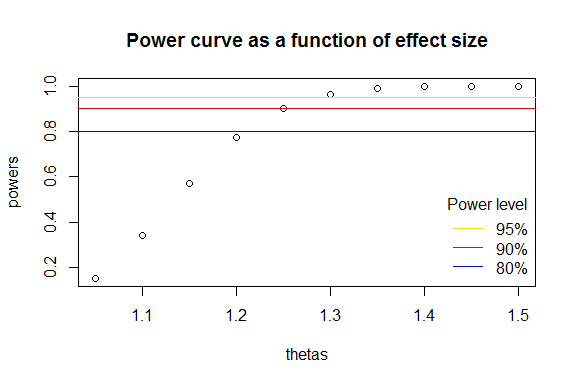# Power Calculation for the Cochran-Mantel-Haenszel Test

## Calculating post hoc Power

### The Data Set

We will use the Titanic dataset in the datasets package to demonstrate a post hoc power calculation. This dataset is explored in greater detail in the introductory vignette.

Let $$X$$ = sex, $$Y$$ = survival, and $$Z$$ = class. Just as was performed in the introductory vignette, we will load the data and then create a set of partial tables stratified at fixed levels of class.

data(Titanic, package = "datasets")
partial_tables <- margin.table(Titanic, c(2,4,1))
ftable(partial_tables)
##                 Class 1st 2nd 3rd Crew
## Sex    Survived
## Male   No             118 154 422  670
##        Yes             62  25  88  192
## Female No               4  13 106    3
##        Yes            141  93  90   20

### The Test Statistic

Performing a CMH test on the partial tables above shows that there is strong evidence that the common odds ratio is greater than one.

mantelhaen.test(partial_tables)
##
##  Mantel-Haenszel chi-squared test with continuity correction
##
## data:  partial_tables
## Mantel-Haenszel X-squared = 360.33, df = 1, p-value < 2.2e-16
## alternative hypothesis: true common odds ratio is not equal to 1
## 95 percent confidence interval:
##   8.232629 14.185153
## sample estimates:
## common odds ratio
##          10.80653

### The Power Calculation

Using the power.cmh.test() function, we may calculate the probability that our rejection of the null hypothesis is a true result.

library(samplesizeCMH)

power.cmh.test(
p1 = apply(partial_tables, 3, prop.table, 2)[1,],
p2 = apply(partial_tables, 3, prop.table, 2)[3,],
N = sum(Titanic),
power = NULL,
s = apply(partial_tables[,1,],2,sum) / apply(partial_tables,3,sum),
t = apply(partial_tables, 3, sum) / sum(Titanic)
)
## NOTE: Effect size ('theta') is not consistent between strata.
## Power and sample size calculation for the Cochran Mantel Haenszel test
##
##                  N = 2201
##        Effective N = 2201
## Significance level = 0.05
##              Power = 1
##        Alternative = two.sided
##
## Number of subjects per each group:
## ______________________________________
## Group   |     1      2      3      4
## ======================================
## Case    |   122    167    528    673
## Control |   203    118    178    212
##
## CALL:
## power.cmh.test(p1 = apply(partial_tables, 3, prop.table, 2)[1,
##     ], p2 = apply(partial_tables, 3, prop.table, 2)[3, ], N = sum(Titanic),
##     power = NULL, s = apply(partial_tables[, 1, ], 2, sum)/apply(partial_tables,
##         3, sum), t = apply(partial_tables, 3, sum)/sum(Titanic))

Perhaps unsurprisingly, the power of this test approaches 100%.

## Power and Effect Size in a Negative Result

It does not make sense to calculate power in a study when the null hypothesis is not rejected. However, it may be of interest to the researcher to determine the minimum effect size that could be detected, given the sample size of the study and a specified power. We use the example of the Nurses’ Health Study explored by Munoz and Rosner (1984). This study looked for a link between oral contraceptives and breast cancer, stratified by age (Barton et al. 1980; Hennekens et al. 1984). The data for this illustration has been included in the samplesizeCMH package.

data(contraceptives, package = "samplesizeCMH")
ftable(contraceptives)
##                            Age Group <= 34 35 - 39 40 - 44 45 - 49 50 - 55
## OC Usage    Disease Status
## Exposed     Case                        31      57      69      86      30
##             Control                    363     521     760     725     272
## Not Exposed Case                        40     107     196     241     132
##             Control                    327    1146    1914    2503    1370

Here, as shown in Hennekens et al. (1984), the Mantel-Haenszel test shows non-significant results, with the common odds ratio approximately equal to 1.

mantelhaen.test(contraceptives)
##
##  Mantel-Haenszel chi-squared test with continuity correction
##
## data:  contraceptives
## Mantel-Haenszel X-squared = 0.33106, df = 1, p-value = 0.565
## alternative hypothesis: true common odds ratio is not equal to 1
## 95 percent confidence interval:
##  0.902928 1.214722
## sample estimates:
## common odds ratio
##          1.047285

The question now is what effect size would be detectable if an effect truly did exist in the data. That is to say, what effect size were we prepared to correctly detect given our data and specified significance level and power? We may vectorize the power.cmh.test() function to test a wide range of effect sizes to see where the effect size crosses over into adequate power.

vpower <- Vectorize(power.cmh.test, "theta", SIMPLIFY = FALSE)

thetas <- seq(1.05,1.5,0.05)

power_list <- vpower(
theta = thetas,
p2 = contraceptives[1,2,] / apply(contraceptives[,2,],2,sum),
N = sum(contraceptives),
power = NULL,
s = 1/11,
t = apply(contraceptives, 3, sum) / sum(contraceptives),
alternative = "greater"
)

powers <- sapply(power_list, "[[", "power")

names(powers) <- thetas

powers
##      1.05       1.1      1.15       1.2      1.25       1.3      1.35
## 0.1507626 0.3399285 0.5718383 0.7734926 0.9027666 0.9659347 0.9901502
##       1.4      1.45       1.5
## 0.9976178 0.9995114 0.9999139

Using the data above, we may create a power curve.

plot(y = powers, x = thetas, main = "Power curve as a function of effect size")
abline(h = 0.95, col = "gold")
abline(h = 0.90, col = "red")
abline(h = 0.80, col = "blue")

legend(
"bottomright",
legend = c("95%", "90%", "80%"),
col = c("gold", "red", "blue"),
bty = "n",
lty = c(1,1),
title = "Power level"
)As we can see from above, 90% power would have been achieved if the common odds ratio was estimated to be approximately 1.25.